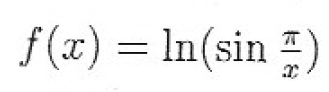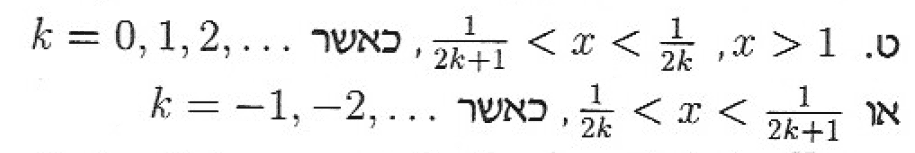# Find the domain of a function of the form: ln(sin(1/x))

• I
sergey_le
TL;DR Summary
I know we need to find out when sin(pi/x)>0.
But I can 't do itthe solutionLast edited by a moderator:

Mentor
2022 Award
If you know the sine function, why can't you say when ##y=\sin x## is positive?

sergey_le
If you know the sine function, why can't you say when ##y=\sin x## is positive?
Because it's not just a sinx.
It's sin(1/x)

Mentor
2022 Award
Because it's not just a sinx.
It's sin(1/x)
Proceed step by step. When is ##y=\sin x > 0##? Which are the intervals of ##x## for which this is true? Let's change the variable names. When is ##Y=\sin X > 0##? If we know that, we get e.g. ##X\in (0,\pi)##.

With that we have now ##X=\dfrac{\pi}{x}##. That is ##0<X=\dfrac{\pi}{x} < \pi##. Can you restructure that in a way, that ##a<x<b## is the result?

sergey_le
Proceed step by step. When is ##y=\sin x > 0##? Which are the intervals of ##x## for which this is true? Let's change the variable names. When is ##Y=\sin X > 0##? If we know that, we get e.g. ##X\in (0,\pi)##.

With that we have now ##X=\dfrac{\pi}{x}##. That is ##0<X=\dfrac{\pi}{x} < \pi##. Can you restructure that in a way, that ##a<x<b## is the result?
I know that sinx> 0 when 0+2πk<x<π+2πk.

I do not understand why it is okay to say that 0+2πk<π/x<π+2πk.

Mentor
2022 Award
I know that sinx> 0 when 0+2πk<x<π+2πk.

I do not understand why it is okay to say that 0+2πk<π/x<π+2πk.
Let us write the sine function as ##\varphi = \sin \alpha##. Then you just said that ##\varphi >0## when ##2k\pi < \alpha < (2k+1)\pi## for ##k\in \mathbb{Z}##. Now how can we compare ##\sin \alpha## and ##\sin (\pi/x)##? We can do this by setting ##\alpha:=\pi/x.## Thus we get from the above inequality that
$$\varphi =\sin \alpha = \sin \left(\dfrac{\pi}{x}\right) > 0 \text{ when } 2k\pi < \alpha = \dfrac{\pi}{x} < (2k+1)\pi \quad (k\in \mathbb{Z})$$
Next question is: Can you transform ##2k\pi < \dfrac{\pi}{x} < (2k+1)\pi## into something like ##A < x < B##?

•sergey_le
sergey_le
Let us write the sine function as ##\varphi = \sin \alpha##. Then you just said that ##\varphi >0## when ##2k\pi < \alpha < (2k+1)\pi## for ##k\in \mathbb{Z}##. Now how can we compare ##\sin \alpha## and ##\sin (\pi/x)##? We can do this by setting ##\alpha:=\pi/x.## Thus we get from the above inequality that
$$\varphi =\sin \alpha = \sin \left(\dfrac{\pi}{x}\right) > 0 \text{ when } 2k\pi < \alpha = \dfrac{\pi}{x} < (2k+1)\pi \quad (k\in \mathbb{Z})$$
Next question is: Can you transform ##2k\pi < \dfrac{\pi}{x} < (2k+1)\pi## into something like ##A < x < B##?
Thank you very much, I finally understood

•berkeman